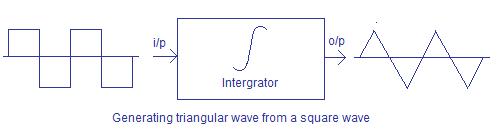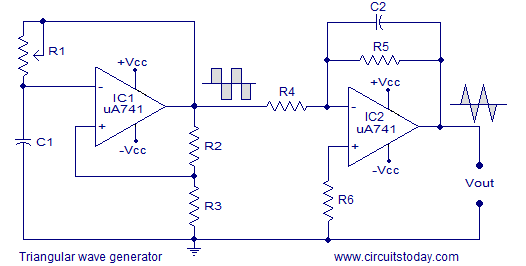## Audio

[Audio][slideshow]

### Triangular wave generator using opamp.

This article is about a triangular wave generator using opamp IC. Triangular wave is a periodic, non-sinusoidal waveform with a triangular shape. People often get confused between triangle and sawtooth waves. The most important feature of a triangular wave is that it has equal rise and fall times while a sawtooth wave has un-equal rise and fall times. The applications of triangular wave include sampling circuits, thyristor firing circuits, frequency generator circuits, tone generator circuits etc. There are many methods for generating triangular waves but here we focus on method using opamps. This circuit is based on the fact that a square wave on integration gives a triangular wave.The circuit uses an opamp based square wave generator for producing the square wave and an opamp based integrator for integrating the square wave. The circuit diagram is shown in the figure below.

#### Circuit diagram.The square wave generator section and the integrator section of the circuit are explained in detail below.

##### Square wave generator.

The square wave generator is based on a uA741 opamp (IC1). Resistor R1 and capacitor C1 determines the frequency of the square wave. Resistor R2 and R3 forms a voltage divider setup which feedbacks a fixed fraction of the output to the non-inverting input of the IC.

Initially, when power is not applied the voltage across the capacitor C1 is 0. When the power supply is switched ON, the C1 starts charging through the resistor R1 and the output of the opamp will be high (+Vcc). A fraction of this high voltage is fed back to the non- inverting pin by the resistor network R2, R3. When the voltage across the charging capacitor is increased to a point the the voltage at the inverting pin is higher than the non-inverting pin, the output of the opamp swings to negative saturation (-Vcc). The capacitor quickly discharges through R1 and starts charging in the negative direction again through R1. Now a fraction of the negative high output (-Vcc) is fed back to the non-inverting pin by the feedback network R2, R3. When the voltage across the capacitor has become so negative that the voltage at the inverting pin is less than the voltage at the non-inverting pin, the output of the opamp swings back to the positive saturation. Now the capacitor discharges trough R1 and starts charging in positive direction. This cycle is repeated over time and the result is a square wave swinging between +Vcc and -Vcc at the output of the opamp.

If the values of R2 and R3 are made equal, then the frequency of the square wave can be expressed using the following equation:

F=1 / (2.1976 R1C1)

##### Integrator.

Next part of the triangular wave generator is the opamp integrator. Instead of using a simple passive RC integrator, an active integrator based on opamp is used here. The opamp IC used in this stage is also uA741 (IC2). Resistor R5 in conjunction with R4 sets the gain of the integrator and resistor R5 in conjunction with C2 sets the bandwidth. The square wave signal is applied to the inverting input of the opamp through the input resistor R4. The opamp integrator part of the circuit is shown in the figure below.Let’s assume the positive side of the square wave is first applied to the integrator. By virtue capacitor C2 offers very low resistance to this sudden shoot in the input and C2 behaves something like a short circuit. The feedback resistor R5 connected in parallel to C2 can be put aside because R5 has almost zero resistance at the moment. A serious amount of current flows through the input resistor R4 and the capacitor C2 bypasses all these current. As a result the inverting input terminal (tagged A) of the opamp behaves like a virtual ground because all the current flowing into it is drained by the capacitor C2. The gain of the entire circuit (Xc2/R4) will be very low and the entire voltage gain of the circuit will be close the zero.

After this initial “kick” the capacitor starts charging and it creates an opposition to the input current flowing through the input resistor R4. The negative feedback compels the opamp to produce a voltage at its out so that it maintains the virual ground at the inverting input. Since the capacitor is charging its impedance Xc keeps increasing and the gain Xc2/R4 also keeps increasing. This results in a ramp at the output of the opamp that increases in a rate proportional to the RC time constant (T=R4C2) and this ramp increases in amplitude until the capacitor is fully charged.

When the input to the integrator (square wave) falls to the negative peak the capacitor quickly discharges through the input resistor R4 and starts charging in the opposite polarity. Now the conditions are reversed and the output of the opamp will be a ramp that is going to the negative side at a rate proportional to the R4R2 time constant. This cycle is repeated and the result will be a triangular waveform at the output of the opamp integrator.

Testing the triangular wave generator.

Testing videos, practical circuit diagram, and photographs will be added soon.

[Lights][grids]